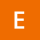Home Communities
IT Knowledge
Inspiration
Languages
EN

Java - calculate difference between two Dates in days

8 points
Created by:Efe-V
259

1. Overview

In java we can calculate difference between two Dates with TimeUnit class.

2. Example of how to calculate difference between two Dates in days

import java.time.LocalDateTime;
import java.time.ZoneOffset;
import java.util.Date;
import java.util.concurrent.TimeUnit;

public class CalculateDifferenceBetweenDays {

public static void main(String[] args) {

LocalDateTime of1 = LocalDateTime.of(2019, 10,  3, 16, 20, 0, 0);
LocalDateTime of2 = LocalDateTime.of(2019, 10, 12, 16, 20, 0, 0);

Date from = Date.from(of1.toInstant(ZoneOffset.UTC));
Date now  = Date.from(of2.toInstant(ZoneOffset.UTC));

long differenceDays = calculateDifferenceBetweenTwoDatesInDays(from, now);

System.out.println("Diff in days: ");
System.out.println(differenceDays); // 9
}

public static long calculateDifferenceBetweenTwoDatesInDays(Date from, Date now) {
long millisecondsDiff = now.getTime() - from.getTime();
return TimeUnit.DAYS.convert(millisecondsDiff, TimeUnit.MILLISECONDS);
}
}

Output:

Diff in days:
9